# Implementing basic Data Structures in Python — Part 1| Daily Python #12

This article is a tutorial that covers the implementation of basic data structures — Linked List, Stack, and Queue. Later articles will cover the remaining data structures.

This article is a part of Daily Python challenge that I have taken up for myself. I will be writing short python articles daily.

Note: This article does not focus on explaining the concept of these basic data structures, the main purpose is to implement these data structures in Python 3.0

`#Implementing basic data structures in Python - LinkedListclass Node:    def __init__(self,value):    self.value = value    self.nextNode = Noneclass LinkedList:  def __init__(self):    self.headNode = None    self.lastNode = None  def insertNode(self,value):    node = Node(value)    if self.headNode == None:      self.headNode = node      self.lastNode = node    else:      self.lastNode.nextNode = node      self.lastNode = node    print(value,'inserted successfully')  def insertNodeAfter(self,value,refValue):    node = Node(value)    if self.headNode == None:      self.headNode = node      self.lastNode = node    else:      iterator = self.headNode      while iterator.value != refValue:        iterator = iterator.nextNode      node.nextNode = iterator.nextNode      iterator.nextNode = node    print(value,'inserted successfully')  def traverseList(self):    iterator = self.headNode    while iterator != None:      print(iterator.value)      iterator = iterator.nextNode  def deleteNode(self,value):    iterator = self.headNode    while iterator.nextNode.value != value:      iterator = iterator.nextNode    temp = iterator.nextNode    iterator.nextNode = temp.nextNode    del(temp)    print(value,' deleted successfully')ll = LinkedList()ll.insertNode(1)ll.insertNode(2)ll.insertNode(3)ll.traverseList()ll.deleteNode(2)ll.traverseList()ll.insertNodeAfter(2,1)ll.traverseList()`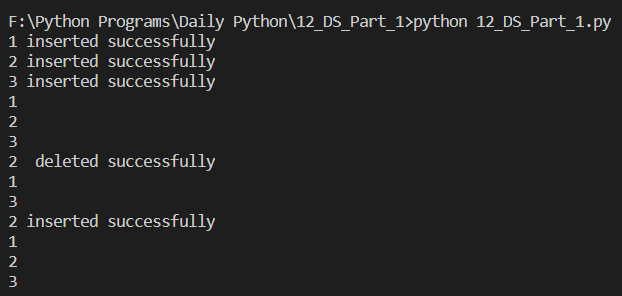Snip of the output of the above code snippet.

The above LinkedList has 2 pointers: headNode and lastNode

For inserting a node at the end of the LinkedList, we simply create a node and then insert it as the ‘nextNode’ of the lastNode

Similarly, for inserting a node in between the LinkedList, we use an iterator to traverse the list and then insert the new node after the given node.

## Now, we’ll proceed with the ‘Stack’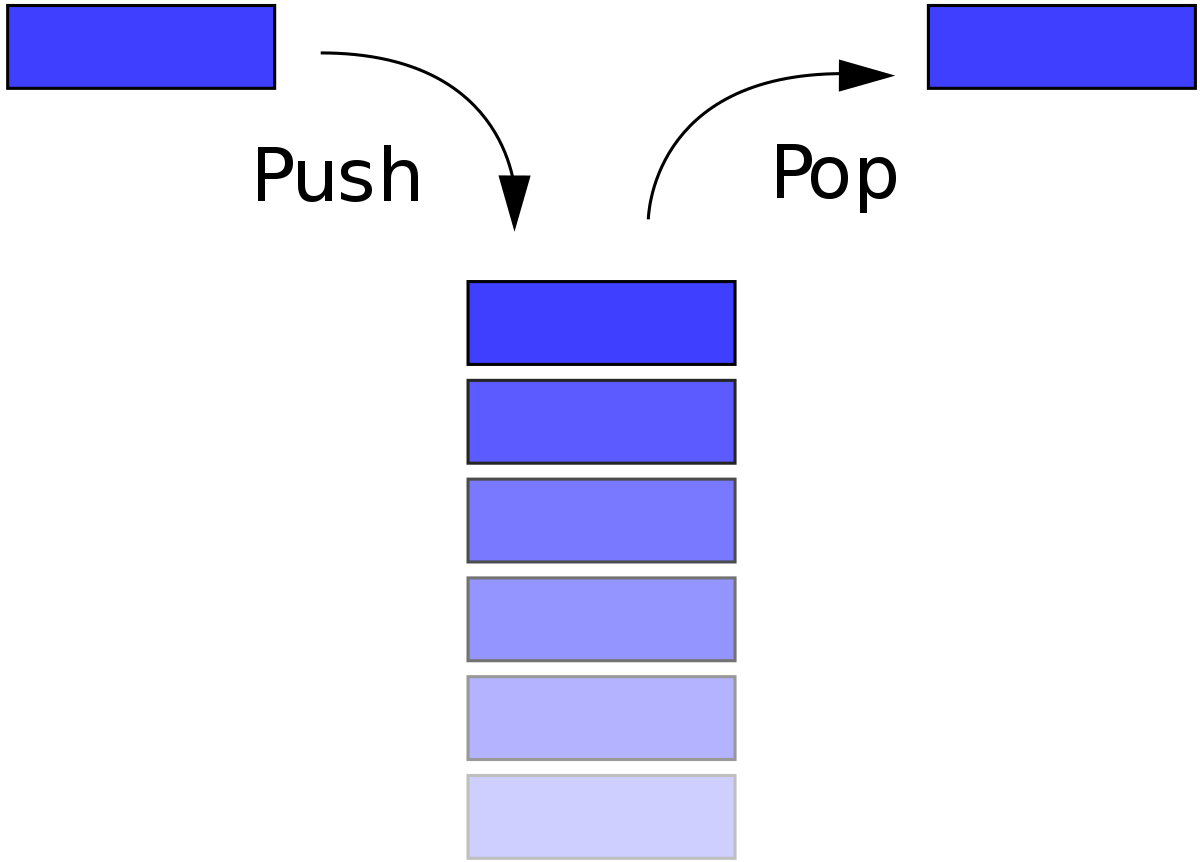Last In First Out — Stack
`#Implementing basic data structures in Python - Stackclass Stack:  def __init__(self):    self.top = -1    self.data = []  def push(self,value):    print(value,' pushed successfully' )        self.data.append(value)    self.top +=1     def pop(self):    if self.top == -1:        print('Stack is empty')                return     print(self.data[self.top],' popped successfully')    del self.data[self.top]    self.top -= 1  def printStack(self):    print('Stack : ',self.data)stack = Stack()stack.push(1)stack.push(2)stack.push(2)stack.printStack()stack.pop()stack.printStack()`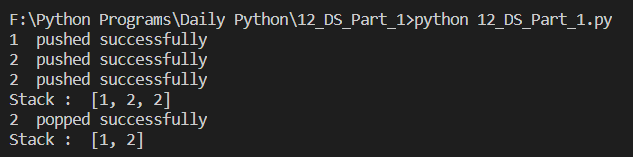Snip of the Output of the above code snippet

## Finally, we see the implementation of the Queue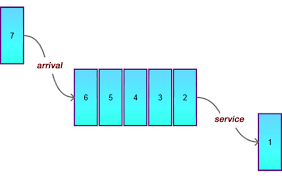First In First Out — Queue
`class Queue:    def __init__(self):        self.front = -1        self.end =  -1        self.data = []        def enqueue(self,value):        print(value,' enqueued successfully')        self.front = 0        self.end += 1        self.data.insert(self.front,value)        def dequeue(self):        if self.end == -1:            print('Queue is empty')                    return        print(self.data[-1],' dequeued successfully')        del self.data[self.end]         self.end -= 1    def printQueue(self):        print('Queue : ',self.data)queue = Queue()queue.enqueue(1)queue.enqueue(2)queue.enqueue(3)queue.printQueue()queue.dequeue()queue.printQueue()`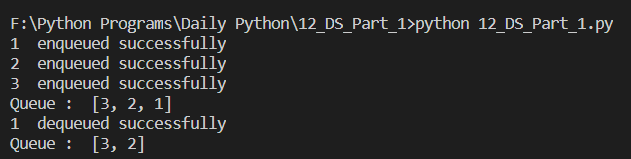Snip of the Output of the above code snippet

I hope this article was helpful, do leave some claps if you liked it.

--

--